# Third Chapter Lesson-14: Logic Circuit from Logic function & Logic function from Logic circuit.

## At the end of this lesson-

• 1. You will be able to implement any function.
• 2. You will be able to determine function from any circuit.

## Implementation of any function:

• The function may need to be implemented with only basic gates.
• The function may need to be implemented with only universal gates.
• The function may need to be implemented using any type of gates.
• After simplifying the function, It may need to be implemented by basic or universal gates.

The following rules or sequences are followed to implement the function using the basic gates:

• If the function needs to be simplified, it needs to be simplified first.
• A common line has to be drawn for each of the variables in the function.
• From the left of the function the basic operations( NOT, AND, OR)  must be done respectively. In this case the parentheses “()” must be given the priority.

Example-1: Implement the following function using only basic gates.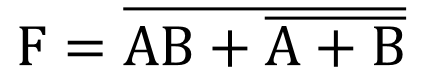Solution:Example-2: Implement the following function.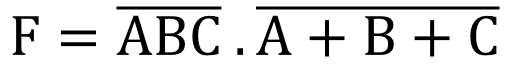Solution: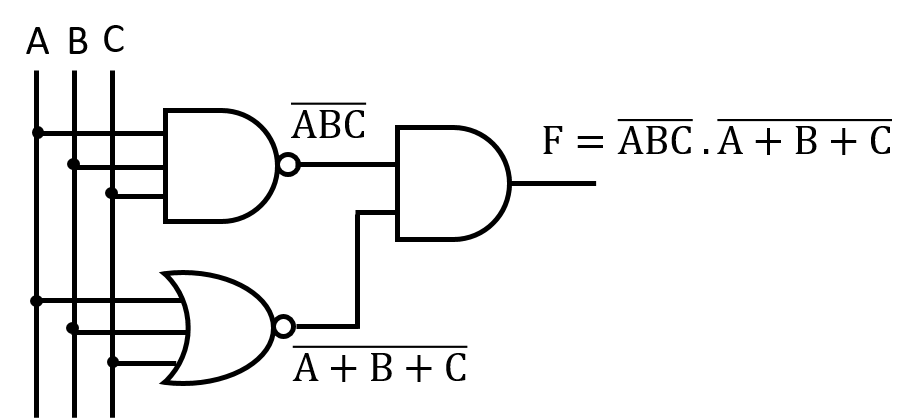## Logic function from Logic Circuit:

• It may need to simplify logic functions from logic circuit.
• Simplified functions from a logic circuit can be implemented by  basic or universal gates.

Example-1: Determine the simplified function from the following circuit.সমাধানঃ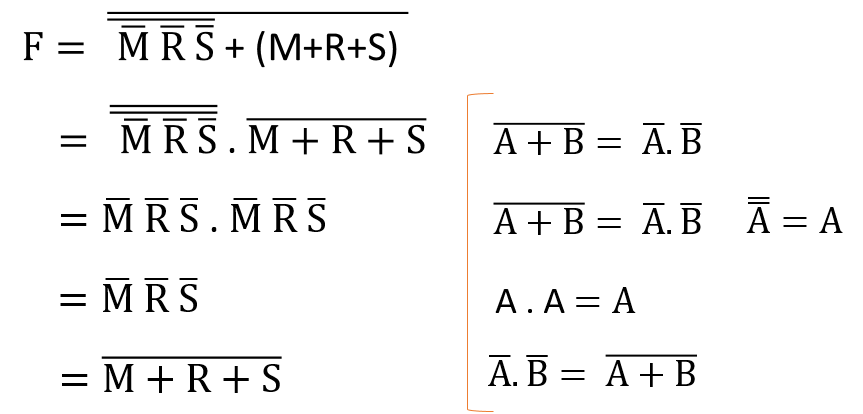Example-2: Implement the simplified function of the following circuit.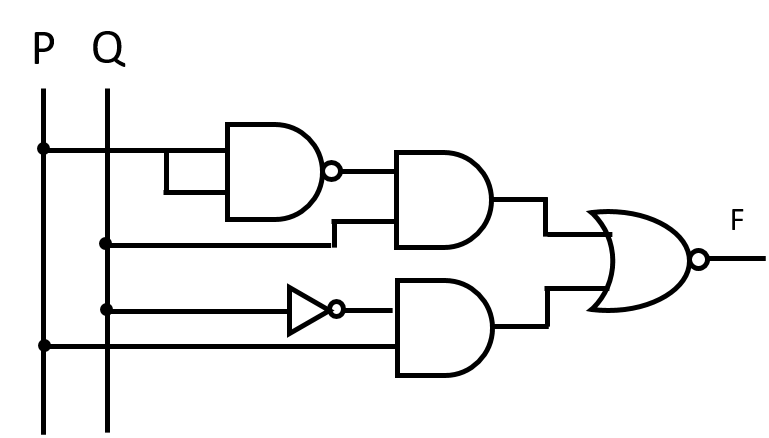সমাধানঃNow implement the above simplified function-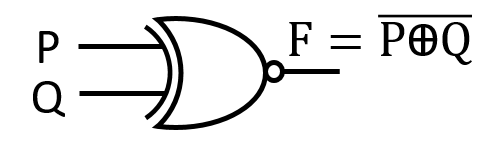## Lesson Evaluation-

Knowledge Based Questions:

Comprehension Based Questions:

Creative Questions:

Multiple Choice Questions:

Written by,